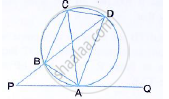Share

# In the Given Figure, Qap is the Tangent at Point a and Pbd is a Straight Line. If ∠Acb = 36° and ∠Apb = 42°, Find: (I) ∠Bap (Ii) ∠Abd (Iii) ∠Qad (Iv) ∠Bcd - Mathematics

Course

#### Question

In the given figure, QAP is the tangent at point A and PBD is a straight line.If ∠ACB = 36° and ∠APB = 42°, find:
(i) ∠BAP (ii) ∠ABD (iii) ∠QAD (iv) ∠BCD

#### Solution

PAQ is a tangent and AB is a chord of the circle.
i) ∴ ∠BAP = ∠ACB  36 (angles in alternate segment)
ii) In ΔAPB
Ext ∠ABD = ∠APB + ∠BAP
⇒ Ext ∠ABD  = 42°  + 36°  = 78°

iii) ∠ADB = ∠ACB = 36°(angles in the same segment)
Ext.  ∠QAD = ∠APB + ∠ADB
⇒ Ext ∠QAD = 42° + 36° = 78°
iv) PAQ is the tangent and AD is chord
∴ QAD =  ∠ACD = 78° (angles in alternate segment)
And ∠BCD = ∠ACB + ∠ACD
∴  ∠BCD = 36° + 78° = 114°

Is there an error in this question or solution?